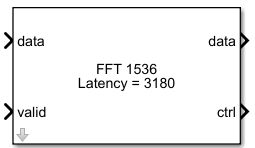# FFT 1536

Computes fast-fourier-transform (FFT) for LTE standard transmission bandwidth of 15 MHz

• Library:
• Wireless HDL Toolbox / Modulation

•## Description

The FFT 1536 block is designed to support LTE standard transmission bandwidth of 15 MHz. This block is used in LTE OFDM Demodulator block operation. The block accepts input data, along with a valid control signal and outputs streaming data with a `samplecontrol` bus.

The block provides an architecture suitable for HDL code generation and hardware deployment.

## Ports

### Input

expand all

Input data, specified as a scalar of real or complex values.

`double` and `single` data types are supported for simulation, but not for HDL code generation.

The more the fractional bits you provide in the input word length, the better the accuracy you receive in the output.

Data Types: `double` | `single` | `int8` | `int16` | `int32` | `fixed point`
Complex Number Support: Yes

Indicates if the input data is valid. When the input valid is `1` (true), the block captures the value on the input data port. When the input valid is `0` (false), the block ignores the input data samples.

Data Types: `Boolean`

When this value is `1` (true), the block stops the current calculation and clears all internal states.

#### Dependencies

To enable this port, select the Enable reset input port parameter.

Data Types: `Boolean`

### Output

expand all

Frequency channel output data, returned as a scalar of real or complex values.

When the input is of `fixed point` data type, the output data type is the same as the input data type. When the input is of integer type, the output data type is of `fixed point` type.

Data Types: `double` | `single` | `int8` | `int16` | `int32` | `fixed point`
Complex Number Support: Yes

Control signals accompanying the sample stream, returned as a `samplecontrol` bus. The bus includes the `start`, `end`, and `valid` control signals, which indicate the boundaries of the frame and the validity of the samples.

• `start` — Indicates the start of the output frame

• `end` — Indicates the end of the output frame

• `valid` — Indicates that the data on the output data port is valid

For more details, see Sample Control Bus.

Data Types: `bus`

## Parameters

expand all

### Main

Specifies the complex multiplier type for HDL implementation. Each multiplication is implemented either with `Use 3 multipliers and 5 adders` or with `Use 4 multipliers and 2 adders`. The implementation speed depends on the synthesis tool and the target device that you use.

Specifies the type of rounding method for internal fixed-point calculations. For more information about rounding methods, see Rounding Modes (DSP System Toolbox). When the input is any integer or fixed-point data type, this block uses fixed-point arithmetic for internal calculations. This parameter does not apply when the input data is `single` or `double`.

When you select this parameter, the block divides the output by 1536. This option is useful when you want the output of the block to stay in the same amplitude range as its input. You require this option when the input is of `fixed point` type.

When you select this parameter, the output word length increases by 2 bits and when you clear this parameter the output word length increases by 11 bits.

### Control Ports

Select this parameter to enable the reset port.

## Algorithms

expand all

To design an FFT 1536 block, radix-3 decimation-in-time (DIT) algorithm is implemented. The input sequence x(n) for all n = {0,1,2....1535} is divided into three DIT sequences, x(3n), x(3n+1), x(3n+2) for all n = {0,1,2....511}.

This equation defines FFT 1536 computation of a given sequence x(n).

`$x\left(k\right)=\sum _{n=0}^{1535}x\left(n\right){W}_{1536}{}^{nk};k=0,1,2,...,1535$`

The equation can be implemented by dividing it into three parts, where P(k), Q(k), R(k) are the N/3 (FFT 512) point FFT of x(3n), x(3n+1), and x(3n+2), respectively. Here, N = 1536, and k = 0,1,2,.....,511.

`$x\left(k\right)=P\left(k\right)+{W}_{N}{}^{k}Q\left(k\right)+{W}_{N}{}^{2k}R\left(k\right)$`
`$x\left(k+N/3\right)=P\left(k\right)+{W}_{3}{}^{1}{W}_{N}{}^{k}Q\left(k\right)+{W}_{3}{}^{2}{W}_{N}{}^{2k}R\left(k\right)$`
`$x\left(k+2N/3\right)=P\left(k\right)+{W}_{3}{}^{2}{W}_{N}{}^{k}Q\left(k\right)+{W}_{3}{}^{1}{W}_{N}{}^{2k}R\left(k\right)$`

This diagram shows the internal architecture of the block and how the input sequence streams through the components of the block.The input sequence x(n) is demultiplexed into three DIT sequences, x(3n), x(3n+1), x(3n+2), each of length 512. Three first-input first-output (FIFO) memories store these sequences. These DIT sequences are serialized and streamed through the FFT 512 block.

## Version History

Introduced in R2019b